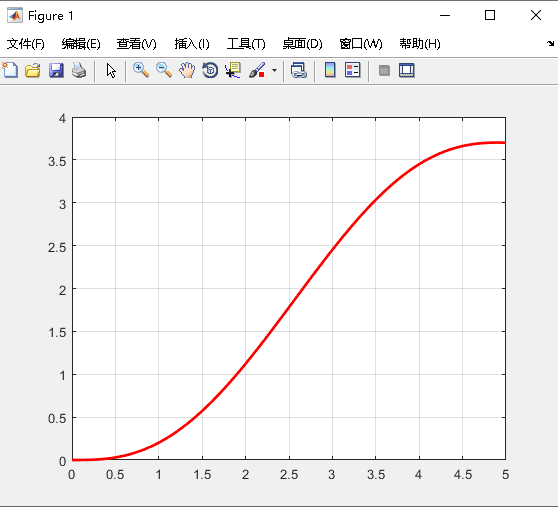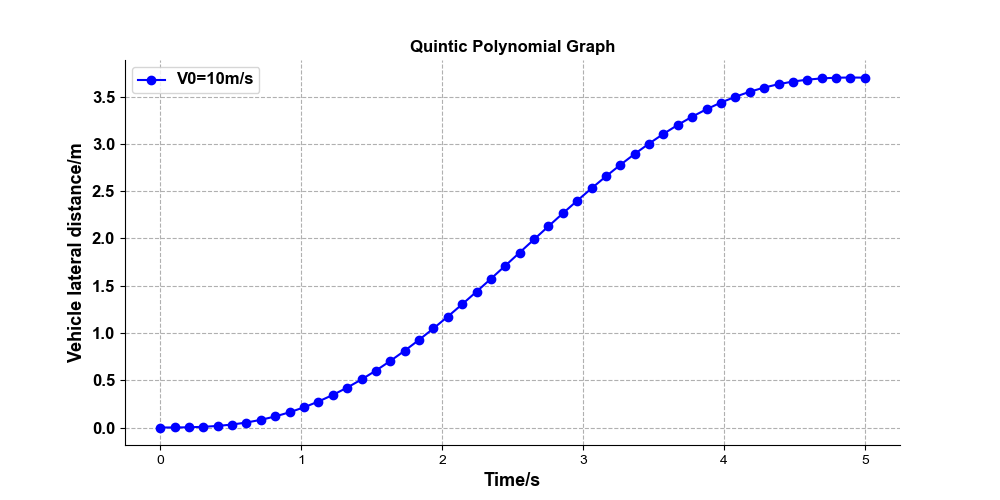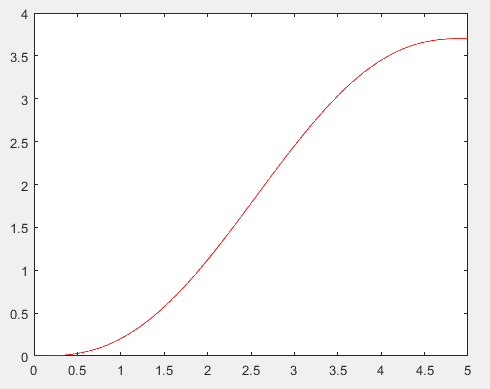• 多项式的表达与创建MATLAB中用一维行向量来表示多项式，将多项式的系数按照降幂次序存放在向量中。请注意上面一句话，这将是MATLAB中对多项式操作的关键MATLAB中对多项式中缺少的幂次的系数应补充为0，不能空过去例...

终于结束了关于MATLAB的基础知识学习部分，开始了对数据的分析

1.多项式的表达与创建

MATLAB中用一维行向量来表示多项式，将多项式的系数按照降幂次序存放在向量中。

请注意上面一句话，这将是MATLAB中对多项式操作的关键

MATLAB中对多项式中缺少的幂次的系数应补充为0，不能空过去

例：输入多项式3x^4 + 23x^3 – 6x +8

Python

>> p = [3 23 0 -6 8]

p =

3 23 0 -6 8

1

2

3

4

5

>>p=[3230-68]

p=

3230-68

2.求根

多项式的根(roots)

例：求例1中多项式的根

Python

>> p = [3 23 0 -6 8]

p =

3 23 0 -6 8

>> r = roots(p)

r =

-7.6263 + 0.0000i

-0.8646 + 0.0000i

0.4121 + 0.4844i

0.4121 - 0.4844i

1

2

3

4

5

6

7

8

9

10

11

12

13

14

>>p=[3230-68]

p=

3230-68

>>r=roots(p)

r=

-7.6263+0.0000i

-0.8646+0.0000i

0.4121+0.4844i

0.4121-0.4844i

由根创建多项式(poly)

Python

>> r = [-7.6263 + 0.0000i; -0.8646 + 0.0000i; 0.4121 + 0.4844i; 0.4121 - 0.4844i];

>> p = poly(r)

p =

1.0000 7.6667 -0.0000 -2.0002 2.6670

1

2

3

4

5

6

>>r=[-7.6263+0.0000i;-0.8646+0.0000i;0.4121+0.4844i;0.4121-0.4844i];

>>p=poly(r)

p=

1.00007.6667-0.0000-2.00022.6670

在这里我们可以看出例子中反求的多项式与原多项式并不一致，其原因在于MATLAB无隙处理复数，当用根重组多项式时，如果一些根有虚部，由于截断误差，则ploy的结果有一些小的虚部。消除虚假的虚部，只要用函数real抽取实部即可

3.多项式的四则运算

加法：c = a+b

乘法：c = conv(a,b)

除法：c = deconv(a,b)

4.导数、积分与估值

导数：b = polyder(a)

积分：b = polyint(a)

估值：h = polyval(g,x)

Python

>> x = -1 : .01 : 1;

>> g = [1 3 5 7 9];

>> h = polyval(g,x);

>> plot(x,h)

1

2

3

4

>>x=-1:.01:1;

>>g=;

>>h=polyval(g,x);

>>plot(x,h)

通过将估值函数与绘图函数结合使用，我们可以方便的看出多项式的值及其值的变化趋势

5.有理多项式

当运算时出现了两个多项式之比的情况时，大多数情况下需要我们将其拆开，即有理化

有理化函数：residue

注：residue函数可进行逆运算

Python

>> num = [5 3 -2 7];

>> den = [-4 0 8 3];

>> [r,p k] = residue(num,den) %num为分子，den为分母

r =

-1.4167

-0.6653

1.3320

p =

1.5737

-1.1644

-0.4093

k =

-1.2500

>> [n ,d] = residue(r,p,k)

n =

-1.2500 -0.7500 0.5000 -1.7500

d =

1.0000 -0.0000 -2.0000 -0.7500

1

2

3

4

5

6

7

8

9

10

11

12

13

14

15

16

17

18

19

20

21

22

23

24

25

26

27

28

29

30

31

32

>>num=[53-27];

>>den=[-4083];

>>[r,pk]=residue(num,den)%num为分子，den为分母

r=

-1.4167

-0.6653

1.3320

p=

1.5737

-1.1644

-0.4093

k=

-1.2500

>>[n,d]=residue(r,p,k)

n=

-1.2500-0.75000.5000-1.7500

d=

1.0000-0.0000-2.0000-0.7500

其中，有理化之后多项式的值为r / (x + p) + k。r、p都可为向量，k为常数

逆有理化之后n表示分母的系数，d表示分子的系数，且分子最高项系数为1

赞赏作者

喜欢 (2)or分享 (0)

展开全文• %产生拟合曲线，并求某点导数% hObject handle to btn_ployder (see GCBO)% event...%显示 set(handles.edit_dy1Value,'string',num2str(dy1Value)); set(handles.edit_dy2Value,'string',num2str(dy2Value));end

%产生拟合曲线，并求某点导数% hObject    handle to btn_ployder (see GCBO)% eventdata  reserved - to be defined in a future version of MATLAB% handles    structure with handles and user data (see GUIDATA)x=0.01:0.01:14;steps=str2num(get(handles.edit_steps,'string')) ;if length(steps)==1%必须是单个曲线        switch get(handles.popupmenu_pick,'value')            case 1                           y=BESSELJ(steps(1),x);                   case 2                y=BESSELY(steps(1),x);                 end        a=polyfit(x,y,str2num(get(handles.edit_polyStep,'string')));%获取拟合多项式系数        a1=polyder(a);%多项式一阶导数        a2=polyder(a1);%多项式二阶导数        t=polyval(a,x);        hold on;        plot(x,y,'b');%绘制贝塞尔曲线        plot(x,t,'r:');%绘制拟合曲线        hold off;                xValue=str2num(get(handles.edit_xValue,'string')) ;        yValue=polyval(a,xValue);%函数值        dy1Value=polyval(a1,xValue);%一阶导数值        dy2Value=polyval(a2,xValue);%二阶导数值                set(handles.edit_yValue,'string',num2str(yValue));%显示        set(handles.edit_dy1Value,'string',num2str(dy1Value));        set(handles.edit_dy2Value,'string',num2str(dy2Value));end

展开全文• （一）使用matlab画多项式函数图像 在用matlab画五次多项式的时候，发现使用y=(0.2771*t^3 - 0.081*t^4 + 0.0063*t^5);总是会报错。搜了搜资料，发现可以这么画： 例如：五次多项式（quintic polynomial） y=0.2771...

（一）使用matlab画多项式函数图像

在用matlab画五次多项式的时候，发现使用y=(0.2771*t^3 - 0.081*t^4 + 0.0063*t^5);总是会报错。搜了搜资料，发现可以这么画：

例如：五次多项式（quintic polynomial） y=0.2771t3-0.081t4+0.0063t5

.m脚本文件

t=0:0.01:5;
p=[0.0063,-0.081,0.2771,0,0,0];
y=polyval(p,t);

figure(1)
plot(t,y,'r','linewidth',2)
grid on
hold on;（二）使用python画多项式函数图像

例如：五次多项式（quintic polynomial） y=0.2771t3-0.081t4+0.0063t5
.py脚本文件

# coding=utf-8
import numpy as np
import matplotlib.pyplot as plt

plt.rcParams['font.sans-serif'] = ['Arial']   # 如果要显示中文字体,则在此处设为：SimHei
plt.rcParams['axes.unicode_minus'] = False    # 显示负号

x = np.linspace(0,5)
y = 0.2771*x*x*x-0.081*x*x*x*x+0.0063*x*x*x*x*x

plt.figure(figsize=(10, 5))
plt.grid(linestyle="--")                      # 设置背景网格线为虚线
ax = plt.gca()
ax.spines['top'].set_visible(False)           # 去掉上边框
ax.spines['right'].set_visible(False)         # 去掉右边框

plt.plot(x, y, marker='o', color="blue",  label="V0=10m/s", linewidth=1.5)
plt.yticks(fontsize=12, fontweight='bold')
plt.title("Quintic Polynomial Graph", fontsize=12, fontweight='bold')
plt.xlabel("Time/s", fontsize=13, fontweight='bold')
plt.ylabel("Vehicle lateral distance/m", fontsize=13, fontweight='bold')

plt.legend(loc=0, numpoints=1)
leg = plt.gca().get_legend()
ltext = leg.get_texts()
plt.setp(ltext, fontsize=12, fontweight='bold')  # 设置图例字体的大小和粗细
plt.show()更新：2020年4月1日
偶然间看到matlab还有一种画多项式函数的方法，而且就是之前报错的那种方法。
.m脚本

t = 0:0.01:5;
y = 0.2771 * t.^3 - 0.081 * t.^4 + 0.0063 * t.^5;  %注意.和^之间没有空格
plot(t, y, 'r')展开全文python matlab 数学建模
• 本篇博客是B站教学视频的学习笔记，...Matlab多项式与数据统计 % 介绍多项式的内容 p=[1,2,3,4]; f1=poly2str(p,'x'); % 生成好看的符号串 % disp(f1) 结果为 x^3 + 2 x^2 + 3 x + 4 f2=poly2sym(p); % 生成可用的符号.

本篇博客是B站教学视频的学习笔记，视频教程地址：https://www.bilibili.com/video/BV1hE411Q7T4，Up主讲的很好，部分内容有删减。部分内容为我自己的理解，由于本人水平有限，可能存在表述不准确的地方，见谅！

Matlab多项式与数据统计

% 介绍多项式的内容
p=[1,2,3,4];
f1=poly2str(p,'x'); % 生成好看的符号串
% disp(f1) 结果为 x^3 + 2 x^2 + 3 x + 4
f2=poly2sym(p); % 生成可用的符号函数
% disp(f2) 结果为 x^3 + 2*x^2 + 3*x + 4
x=2;
y=polyval(p,x); %带入求值，即求 x^3 + 2*x^2 + 3*x + 4 在x=2时的值。
% 知道系数矩阵，求根
r=roots(p);
% 知道根，求系数矩阵
p_=poly(r);
% 数据插值操作
X=[-3,-1,0,1,3];
Y=[9,1,0,1,9];
% 上面两行描述已知点。
y2=interp1(X,Y,2); % 使用插值方法，预测当x=2时，值为多少
y2m=interp1(X,Y,2,'spline'); %使用三次样条方法插值，估计当x=2时的值为多少。

% 数据统计操作
% 定义数据
X = [2, 3, 9, 15, 6, 7, 4];
A = [1, 7, 2; 9, 5, 3; 8, 4 ,6];
B = [1, 7, 3; 9, 5, 3; 8, 4 ,6];

% 求矩阵的最大值
y=max(X);
[y,k]=max(X); % k为最大值的角标，y为最大值的值
max(A,[],1); % 求每一列的最大值，结果为行向量
max(A,[],2); % 求每一行的最大值，结果为列向量,
[y,k]=max(A,[],1); % 不仅返回最大值，还有角标位置

% 均值和中值
y=mean(X);
mean(A,1); % 求Ａ每一列的均值，结果为行向量
mean(A,2); % 求Ａ每一行的均值，结果为列向量
y=median(X);
median(A,1); % 求A每一列的中值，结果为行向量
median(A,2); % 求A每一行的中值，结果为列向量

% 其它运算
y = sum(X); % 求和
y = prod(X); % 求积
y = cumsum(X); % 累加
y = cumprod(X); % 累乘

% 排序操作
% sort(矩阵, dim, 'method') dim为1按列排序，2按行排序；
% ascend升序，descend降序
Y = sort(A, 1, 'ascend');
[Y,I] = sort(A, 1, 'ascend'); % I表示Y中对应元素在原来数组中的角标

Matlab符号函数

% 符号函数创建
% 使用sym函数
p=sin(pi/3); % p = 0.8660
% p:符号常量
% 第二个参数：d:浮点数，f:有理分数，e:有理数和误差，r:有理数
P=sym(p,'r'); % 用数值创建符号常量P；

%使用syms函数
syms x; %声明符号变量
f=7*x^2+2*x+9; %创建符号函数

% 符号运算
%符号运算
% 加减乘除外
% '转置 ； ==相等 ； ~=不等
% sin, cos, tan; asin, acos, atan 三角反三角
% sinh, cosh, tanh; asinh, acosh, atanh 双曲反双曲
% conj复数共轭；real复数实部；imag复数虚部；abs复数模；angle复数幅角
% diag矩阵对角；triu矩阵上三角；tril矩阵下三角；inv逆矩阵；det行列式；rank秩；poly特征多项式；
% |----expm矩阵指数函数；eig矩阵特征值和特征向量；svd奇异值分解；

% 精度控制
digits; % 显示当前计算精度
digits(16); % 将精度设置为16
a16=vpa(sqrt(2)); % 以16位的精度计算sqrt(2);
a8=vpa(sqrt(2),8); % 以8位的精度计算sqrt(2);

% 符号多项式函数运算
g=expand(f); % 展开
h=collect(g); % 整理，默认按照x整理
h1=collect(f,x); % 按照x整理；
% 因式分解展开质因数
fac=factor(h);
factor(12);
% 符号多项式向量形式与计算
syms a b c; % 定义符号变量
n=[a,b,c]; % 构建符号向量
roots(n);  % 求符号多项式ax^2+b^x+c=0的根
n=[1,2,3];
roots(n); % 求符号多项式带入 a=1,b=2,c=3的根
% 反函数
fi=finverse(f,x);

% 符号函数微积分
limit(f,x,4); % 求关于x的函数在x->4时的极限
limit(f,4); % 默认变量等于4时的极限
limit(f); % 等价于 limit(f,0)
limit(f,x,4,'left'); % 求左极限，x->4-

% 基本级数运算
syms x;
s=x+1;
symsum(s,x,[0 2]); % 结果是6，分别将0,1,2 带入x+1并求和
symsum(s,x,0,2); %与上面等价
symsum(s,x,[0;2]) % 与上面等价
% 一维泰勒展开
taylor(f,x,4); % 在x=4处展开为5阶泰勒级数
taylor(f,4); % 在默认变量=4处展开为5阶泰勒级数
taylor(f); % 在默认变量=0处展开为5阶泰勒级数

% 符号微分
n=1;
fn=diff(f,x,n); % f(x) 对x的n阶导
diff(f,1);
diff(f);

% 多元偏导
fxy=diff(f,x,y); % 先求x偏导，再求y偏导
fxyz=diff(f,x,y,z); % 先求x偏导，再求y偏导，最后求z偏导

% 符号积分
% 定积分
int(s,x,1,2);
int(s,1,2);
% 不定积分
int(s,x);
int(s);

% 符号方程求解
% 一元方程
eqn1=a*x==b;
S=solve(eqn1);
% 多元方程组
eqn21 = x-y==a;
eqn22 = 2*x+y==b;
% [Svar1,...SvarN]=solve(eqn1,...eqnM, var1,...varN),MN不一定相等
[Sx, Sy] = solve(eqn21, eqn22, x, y);
% 加上参数ReturnCondition可返回通解及解的条件
[Sxn, Syn] = solve(eqn21, eqn22, x, y, 'ReturnCondition', true);
% 其他参数(参数加上true生效)
% IgnoreProperty，忽略变量定义时一些假设
% IgnoreAnalyticConstraints，忽略分析限制；
% MaxDegree，大于3解显性解；
% PrincipleValue，仅主值
% Real，仅实数解

展开全文• 编写多项式函数的参数辨识系统，多项式函数的形式为：y = a0 + a1·x + a2·x2 + …+ an·xn式中，x为自变量 （xmin≤x≤xmax），y为因变量，a0, a1, a2, …, an为多项式的系 数，n为多项式的次数。 每个...c++ 数学
• pyplot绘制多项式函数图

千次阅读 2018-06-24 14:41:34
pyplot绘制多项式函数图 # -*- coding: utf-8 -*- """ Created on Sun Jun 24 14:33:10 2018 @author: muli """ import numpy as np import matplotlib.pyplot as plt # 以...
• PCLPlotter显示多项式函数1.版本要求2.简介3.代码4.效果 1.版本要求 版本: >PCL1.7 2.简介 PCLPlotter是PCL开源库中应用绘制图形的类，可以绘制多项式函数和直方图。PCLPlotter简便易用，本例子是使用PCLPlotter...
• 多项式拟合。 然后画出函数曲线来。以及给出函数形式。 求导。 再画出导数的曲线。以及给出导数函数形式。 matlab代码： clc close all clear %% 给出一列数 xx = 1:100; yy = xx.^3 - xx.^2; first = 1; ...matlab 多项式拟合 线性拟合
• MATLAB多项式函数及方程求根及绘图

千次阅读 多人点赞 2020-04-29 01:26:40
多项式函数 多项式的构造 向量 [ a , b , c , d ] 表示的多项式为： poly函数的两种用法： P = ploy(r)：以向量 r 内的元素为根，返回多项式的系数 P = ploy(A)：返回矩阵 A 的特征多项式 如果紧接着 roots(P)...
• 最小二乘法实现N次多项式函数拟合 最近都在全力准备电赛，无奈由于郑州的疫情，整个河南赛区和其他一起赛区推迟了全国电子设计大赛，于是，闲暇之中和大家分享一下，使用最小二乘法来实现N次多项式的拟合函数。 新的...c语言 过拟合 stm32 单片机
• 小作业，大想法：Python计算多项式函数 偶然中接到了一项小作业，初看很简单，思路基本在脑中了，但是当我打开Pycharm后打了第一次，却显示结果不对，那说明逻辑错误了。 先来看看题吧： 编一个函数，计算多项式的...
• sklearn生成多项式import numpy as npfrom sklearn.preprocessing import polynomialfeatures #这哥用于生成多项式x=np.arange(6).reshape(3,2) #生成三行二列数组reg = polynomialfeatures(degree=3) #这个3看下面...
• 多项式函数拟合实验 注：t.my_ones_packages是我自己根据《动手学深度学习》目前学习过程中出现的所有自定义函数进行集中得到的文档。 《动手学深度学习》也向读者提供了一个包含所有自定义函数的包“d2lzh”大家...深度学习 多项式函数拟合 过拟合 欠拟合
• 是否有任何python函数(可能来自numpy或scipy)计算扩展中x ** r的系数(1 xx ** 2 x ** 3 … x **(k-1))** n ,其中k> = 1,n> = 0且0 <= r <= n(k-1)？这有时被称为多项式系数(PC)(参见,例如,here).如果...
• # -*- coding: utf-8 -*- """ Created on Sun Jun 2 11:12:40 2019 @author: User """ # -*- coding: utf-8 -*- """ Created on Sat Jun 1 23:18:28 2019 @author: User """ ...import matplo...
• sym2str.m 返回一个字符串从符号多项式表示多项式matlab
• 多项式拟合： x=1:10; y=randn(size(x)); p=polyfit(x,y,7); % 7阶多项式，返回拟合多项式的降幂系数 x1=0:.1:10; y_hat=polyval(p,x1); plot(x1,y_hat,x,y,'r*') 或者直接使用matlab的拟合工具箱 写好x, y值 x=1:...
• 此外，该函数显示两个数字，第一个显示根与权重，第二个显示对应的正交多项式，直到指定的阶数 m。 最后，可以看出由于权重公式的一般实现，其他正交多项式可以很容易地包含在函数中（case ...）。 --------------...matlab
• 函数要么在圆形、六边形、椭圆形、矩形或环形光瞳上显示泽尼克多项式，要么将表面数据拟合到这些光瞳形状。 见 Mahajan, VN, G.-m。 Dai，“波前分析中的正交多项式：解析解”，J. Opt。 社会。 是。 A，卷。 2007...matlab
• 多项式运算

2017-11-04 21:21:14
(2)打印显示多项式；(3)销毁多项式；(4)复制多项式；(5)求两个多项式的和；(6)求两个多项式的差；(7)求两个多项式的积；(8)求一个多项式的n次幂；(9)计算一个多项式在x=a处的值。 高级功能：(1)求一个多项式的n阶导...多项式
• %功能：本程序为多项式拟合，输出多项式表达式并求值 %说明：x,y为插值节点和节点上的函数值，n是多项式最高项次数，xx是所求函数值的x值 %说明：x,y,xx都可以是向量，n是数字 %实例：在命令行键入：Polyfit_Valve(...MATLAB 多项式拟合
• 1. 原始数据：假如要拟合的数据yyy来自sin函数，np.sinimport numpy as npimport matplotlib.pyplot as pltxxx = np.arange(0, 1000) # x值，此时表示弧度yyy = np.sin(xxx*np.pi/180) #函数值，...
• 1多项式的表示 2程序框架 3读多项式 4加法实现 5乘法实现 6输出多项式 程序代码如下： #include #include /* 定义结构，但是更推荐这种定义 typedef struct PolyNode{ int coef; int expon; struc
• python numpy 多项式拟合 """ 多项式拟合 """ import numpy as np ...# 方法-日期转换函数 def dmy2ymd(dmy): dmy = str(dmy, encoding='utf-8') time = dt.datetime.strptime(dmy, '%d-%m-%Y'
• 目标8：Simulink求解传递函数 启动Simulink： 一般人我都不告诉的绝招之一： 一堆模块需要连线，例如前...目标1： 显示多项式传递函数 分子和分母各项系数按照降次排列，分别计入 num=[ ], den=[], 缺项系数补零。 ...
• 此外，系统会显示函数在显示域上的积分值。 如果函数发散，则将显示无穷大符号。 图形的比例可以使用 FPGA 中的 LED 来确定。 Xilinx ISE 用于编写VHDL 文件并合成相应的project.bit，可以直接传输到合适的FPGA 以...
• matlab多项式及其运算

万次阅读 多人点赞 2018-11-17 21:19:00
matlab中，提供了poly2sym函数实现多项式的构造。 r = poly2sym(c)：c为多项式的系数向量 r = poly2sym(c, v)：c为多项式的系数向量，v为其变量 &gt;&gt; poly2sym([1 3 2]) ans = x^2 + 3*x + 2 &...matlab 多项式 基础 语言 笔记
• 全域多项式插值指的是在整个插值区域内形成一个多项式函数作为插值函数。关于多项式插值的基本知识，见“计算基本理论”。 　在单项式基插值和牛顿插值形成的表达式中，求该表达式在某一点处的值使用的Horner嵌套...
• MATLAB多项式

2021-04-21 23:43:23
MATLAB指多项式行向量...计算多项式polyval 函数用于将指定的值 - 计算多项式。例如，要计算我们以前的多项式p, x = 4, 输入:p = [1 7 0 -5 9];polyval(p,4)MATLAB 执行上面的语句，并返回以下结果：ans = 693MAT......